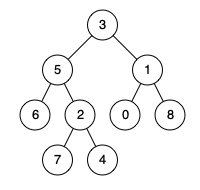236. Lowest Common Ancestor of a Binary Tree
Medium
14.1K
331

Given a binary tree, find the lowest common ancestor (LCA) of two given nodes in the tree.

According to the definition of LCA on Wikipedia: “The lowest common ancestor is defined between two nodes `p` and `q` as the lowest node in `T` that has both `p` and `q` as descendants (where we allow a node to be a descendant of itself).”

Example 1:```Input: root = [3,5,1,6,2,0,8,null,null,7,4], p = 5, q = 1
Output: 3
Explanation: The LCA of nodes 5 and 1 is 3.
```

Example 2:```Input: root = [3,5,1,6,2,0,8,null,null,7,4], p = 5, q = 4
Output: 5
Explanation: The LCA of nodes 5 and 4 is 5, since a node can be a descendant of itself according to the LCA definition.
```

Example 3:

```Input: root = [1,2], p = 1, q = 2
Output: 1
```

Constraints:

• The number of nodes in the tree is in the range `[2, 105]`.
• `-109 <= Node.val <= 109`
• All `Node.val` are unique.
• `p != q`
• `p` and `q` will exist in the tree.
Accepted
1.3M
Submissions
2.2M
Acceptance Rate
59.1%

Seen this question in a real interview before?
1/4
Yes
No

Discussion (0)

Related Topics